# For the reaction N2 (g) + 3H2(g) --> 2 NH3 (g), (a) what is the reaction...

For the reaction N2 (g) + 3H2(g) --> 2 NH3 (g),

(a) what is the reaction Gibbs free energy at equilibrium in J/mol?

The equilibrium constant of the reaction N2 (g) + 3H2(g) --> 2 NH3 (g) at 81 oC is Keq = 478,789.

(b) What is the standard reaction Gibbs energy of this reaction in J/mol?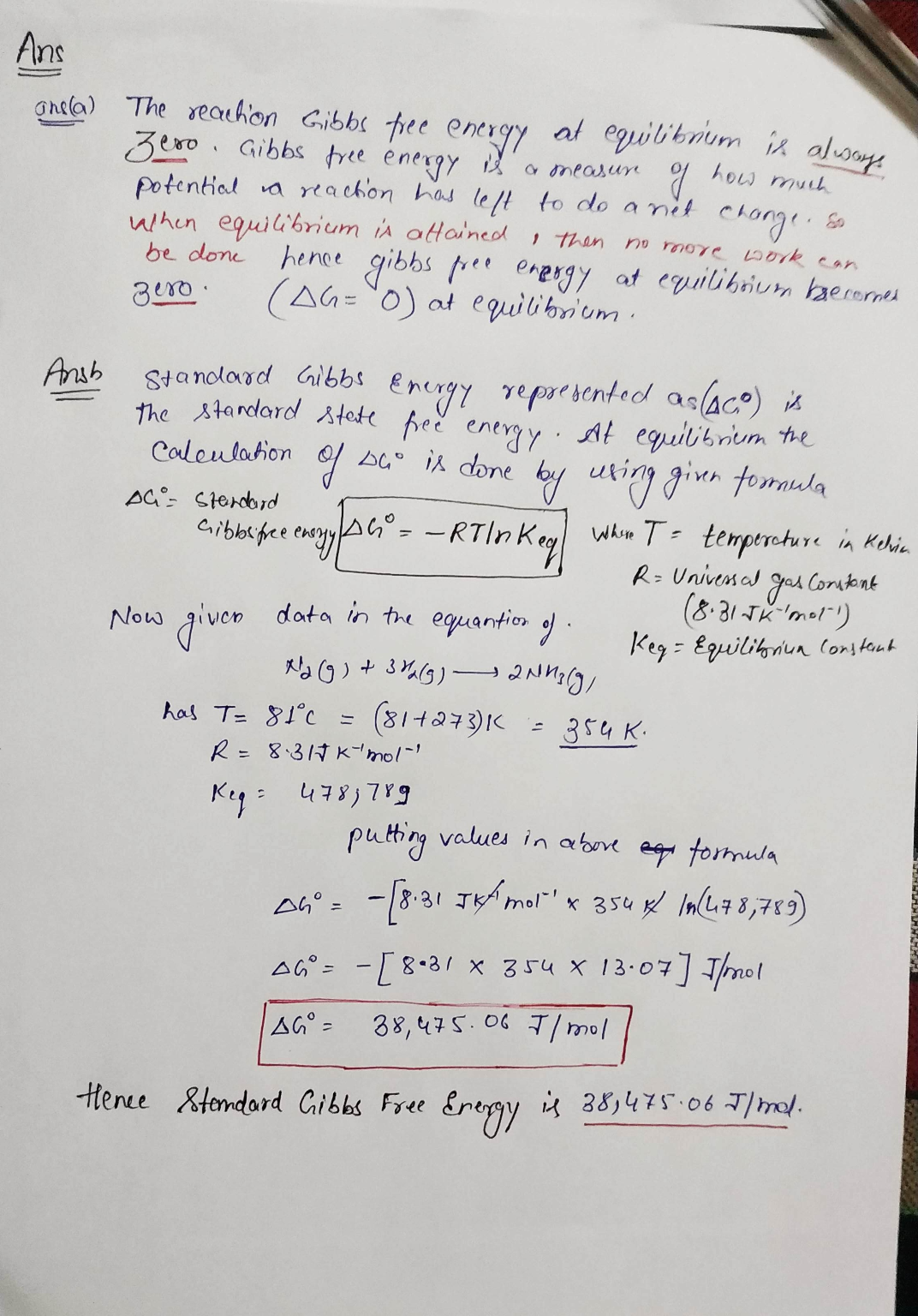##### Add Answer of: For the reaction N2 (g) + 3H2(g) --> 2 NH3 (g), (a) what is the reaction...
Similar Homework Help Questions
• ### 1. The simplest ammonia formation is from nitrogen and hydrogen. Consider the reversible reaction N2(g) + 3H2(g) → 2NH3(g) The standard enthaply and Gibbs free energy of formation one mole NH3 is ∆H◦...

1. The simplest ammonia formation is from nitrogen and hydrogen. Consider the reversible reaction N2(g) + 3H2(g) → 2NH3(g) The standard enthaply and Gibbs free energy of formation one mole NH3 is ∆H◦ m = −46.11 kJ mol−1 and ∆G◦ m = −16.78 kJ mol−1 . (a) What is equilibrium constant at standard condition (25°C and 1 atm)? (b) What is equilibrium constant at 60°C and 1 atm? (c) What is the Gibbs free-energy change relative to that under standard...

• ### 2. (Type III) The reaction N2(g) + 3H2(g) → 2 NH3(g) starts with 6.00 moles of...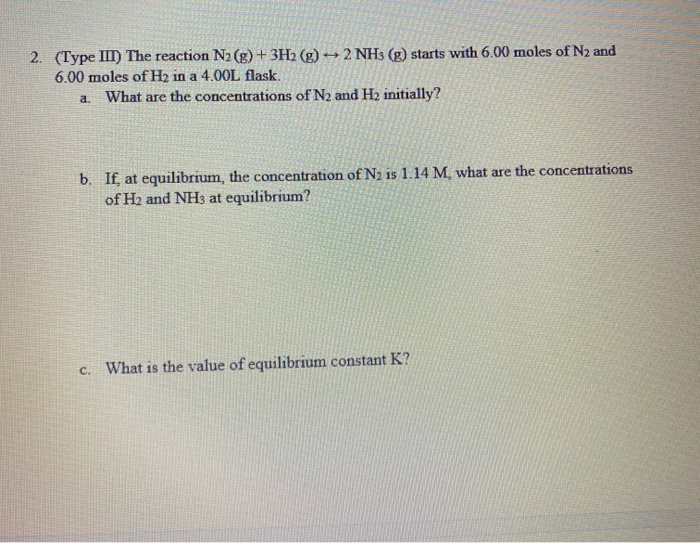2. (Type III) The reaction N2(g) + 3H2(g) → 2 NH3(g) starts with 6.00 moles of N2 and 6.00 moles of H2 in a 4.00L flask. a. What are the concentrations of N2 and H2 initially? b. If, at equilibrium, the concentration of N2 is 1.14 M. what are the concentrations of H2 and NH3 at equilibrium? c. What is the value of equilibrium constant K?

• ### The equilibrium constant, K, for the following reaction is 6.30 at 723K. 2NH3(g) N2(g) + 3H2(g)...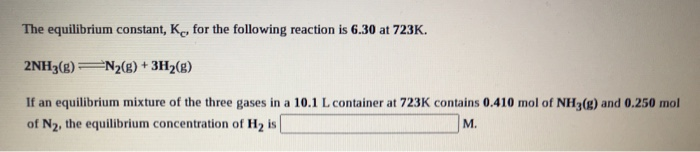The equilibrium constant, K, for the following reaction is 6.30 at 723K. 2NH3(g) N2(g) + 3H2(g) If an equilibrium mixture of the three gases in a 10.1 L container at 723K contains 0.410 mol of NH3(g) and 0.250 mol of N2, the equilibrium concentration of Hy is M.

• ### 3. Consider the ammonia production reaction, N2(g)+3H2(g) = 2NH3 (g) The equi librium constant for this reaction at 298...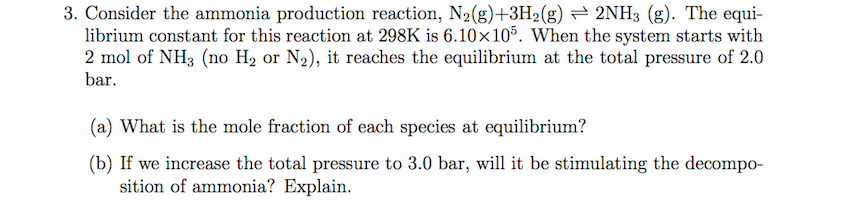3. Consider the ammonia production reaction, N2(g)+3H2(g) = 2NH3 (g) The equi librium constant for this reaction at 298K is 6.10x 105. When the system starts with 2 mol of NH3 (no H2 or N2), it reaches the equilibrium at the total pressure of 2.0 bar (a) What is the mole fraction of each species at equilibrium? (b) If we increase the total pressure to 3.0 bar, will it be stimulating the decompo- sition of ammonia? Explain

• ### 3. Consider the ammonia production reaction, N2(g)+3H2(g) + 2NH3 (g). The equi- librium constant for this...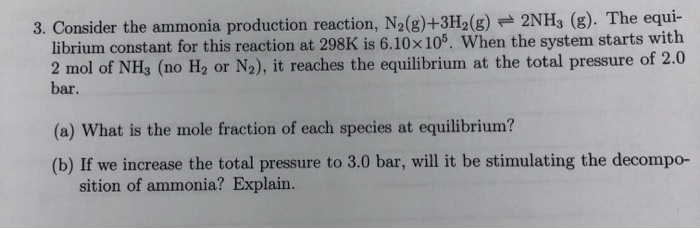3. Consider the ammonia production reaction, N2(g)+3H2(g) + 2NH3 (g). The equi- librium constant for this reaction at 298K is 6.10 x 105. When the system starts with 2 mol of NH3 (no H, or N2), it reaches the equilibrium at the total pressure of 2.0 bar. (a) What is the mole fraction of each species at equilibrium? (b) If we increase the total pressure to 3.0 bar, will it be stimulating the decompo- sition of ammonia? Explain.

• ### Question 5 0/1 point The equilibrium constant for the gas phase reaction N2 (g) + 3H2(g)...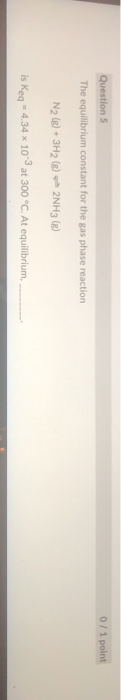Question 5 0/1 point The equilibrium constant for the gas phase reaction N2 (g) + 3H2(g) + 2NH3 (B) is Keq - 4,34 x 10-3 at 300 °C. At equilibrium, Question 5 The equilibrium constant for the gas phase reaction N2 (8) + 3H2 (g) – 2N63 (g) is keq = 4.34 x 10-3 at 300 °C. At equilibrium, products predominate only reactants are present roughly equal amounts of products and reactants are present only products are present reactants predominate

• ### 4. Hydrogen reacts with nitrogen to form ammonia (NH3) according to the reaction 3H2(g) + N2(g)...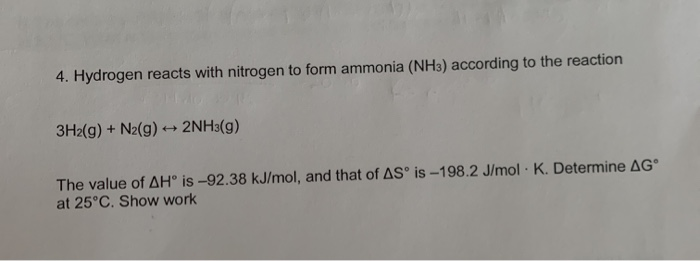4. Hydrogen reacts with nitrogen to form ammonia (NH3) according to the reaction 3H2(g) + N2(g) + 2NH3(g) The value of AH is -92.38 kJ/mol, and that of AS is -198.2 J/mol · K. Determine AGⓇ at 25°C. Show work

• ### Ammonia (NH3) is formed via the following reaction between hydrogen (H2) and nitrogen (N2): 3H2(g) +...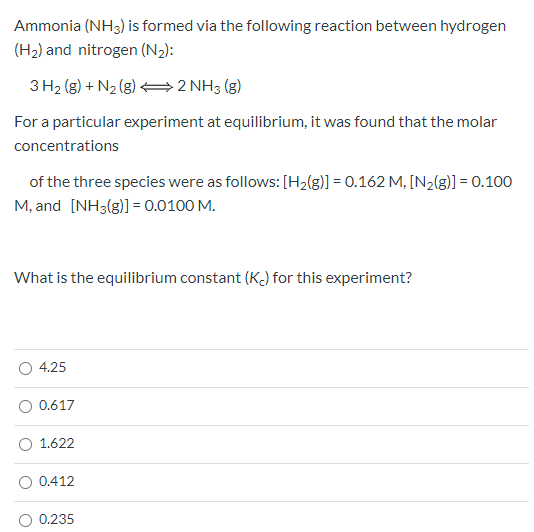Ammonia (NH3) is formed via the following reaction between hydrogen (H2) and nitrogen (N2): 3H2(g) + N2(g) 22 NH3(g) For a particular experiment at equilibrium, it was found that the molar concentrations of the three species were as follows: [H2(g)] = 0.162 M, [N2(g)] = 0.100 M. and [NH3(g)] = 0.0100 M. What is the equilibrium constant (K) for this experiment? 4.25 0.617 1.622 0.412 0.235

• ### uton containing 110 5. Determine the equilibrium constant Kp at 25°C for the reaction N2(g) + 3H2(g) [AG°f (NH3(g))...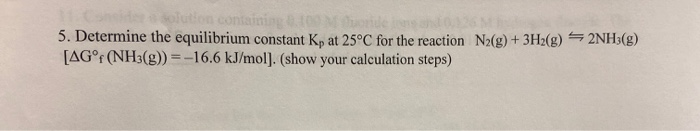uton containing 110 5. Determine the equilibrium constant Kp at 25°C for the reaction N2(g) + 3H2(g) [AG°f (NH3(g)) = -16.6 kJ/mol]. (show your calculation steps) de 2NH3(g)

• ### For the reaction N2(g) + 3H2(g) = 2 NH3(g), what is AG (in kJ) at 298...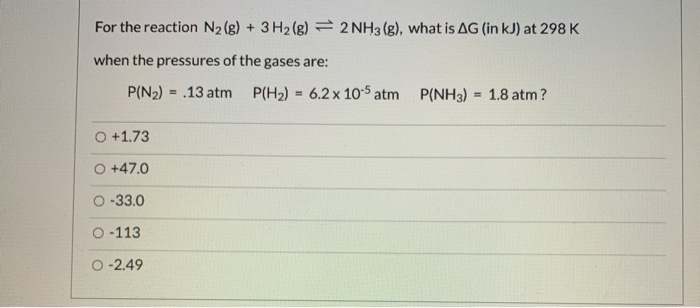For the reaction N2(g) + 3H2(g) = 2 NH3(g), what is AG (in kJ) at 298 K when the pressures of the gases are: P(N2) = .13 atm P(H2) = 6.2 x 10-5 atm P(NH3) = 1.8 atm? +1.73 +47.0 0 -33.0 0-113 0 -2.49 Show transcribed image text

Free Homework App